Question

An Accountant using the expressions (4a+3b-6c) and (5a+c) to calculate the total compensation for employees in two different departments of a business.

What is the sum of the two expressions?​

1.kimchi2

The sum of two expressions will be:

•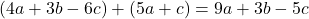Step-by-step explanation:

Given the expressions

•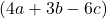•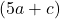Let us calculate the sum of two expressions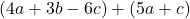Remove the parentheses: (a)=a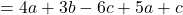Group like terms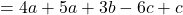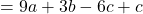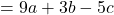•